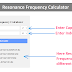Here, using this Resonant Frequency Calculator you can calculate the resonant frequency by providing the inductance and capacitance values. This calculator allows you to perform calculations with different units from a very small unit(Pico) to a very large unit(Giga).

Resonant Frequency Calculator

## Resonant Frequency Calculator

### How to Use This Calculator?

To use the above "Resonant Frequency Calculator," follow the below steps,

1. In the first input box labeled "Capacitance," enter the value of the capacitance you want to calculate the resonant frequency for.

3. In the second input box labeled "Inductance," enter the value of the inductance you want to calculate the resonant frequency for.

4. Choose the appropriate unit for the inductance from the dropdown menu next to the input box. The available units are picohenries (pH), nanohenries (nH), microhenries (uH), millihenries (mH), henries (H), kilohenries (kH), megahenries (MH), and gigahenries (GH).
Click "Calculate":

5. After entering the capacitance and inductance values, click on the "Calculate" button below the input fields.

6. The calculated resonant frequency will be displayed in the result section.

Please note that the resonant frequency is calculated based on the capacitance and inductance values provided, and the result will be shown in the same unit as the frequency. Ensure that you input the values correctly and select the appropriate units for accurate calculations.

### Formula

Here is the formula for calculating the Resonant Frequency,

Resonant Frequency (f0) = 1 / (2 * π * √(L * C))

Where:

f0 is the resonant frequency in Hertz (Hz).
L is the inductance of the inductor in Henrys (H).
C is the capacitance of the capacitor in Farads (F).
π (pi) is a mathematical constant approximately equal to 3.14159.

### Example

Let's take an example to calculate the resonant frequency.

Suppose we have the following parameters:

Inductance (L) = 100 millihenries (mH)
Capacitance (C) = 1 microfarad (uF)

Now, we can calculate the resonant frequency (f0) using the formula:

Resonant Frequency (f0) = 1 / (2 * π * √(L * C))

First of all, convert the values to base units:

Inductance (L) = 100 millihenries (mH) = 0.1 H
Capacitance (C) = 1 microfarad (uF) = 1e-6 F

Putting the values into the formula:

f0 = 1 / (2 * π * √(0.1 * 1e-6))

Calculate the value of 2 * π:

2 * π ≈ 6.2832

Calculate the square root of (0.1 * 1e-6):

√(0.1 * 1e-6) ≈ 0.0003162

Continue with the calculation:

f0 = 1 / (2 * π * 0.0003162)

Finally, calculate the resonant frequency (f0):

f0 ≈ 1 / (6.2832 * 0.0003162) ≈ 500.65 Hz

So, in this example, the resonant frequency is approximately 500.65 Hz. This means when the circuit is excited with a sinusoidal voltage at 500.65 Hz, it will show the resonance property.

Thank you for visiting the Website. Keep visiting for more Updates.

Resonant Frequency CalculatorReviewed by Author on August 04, 2023 Rating: 5